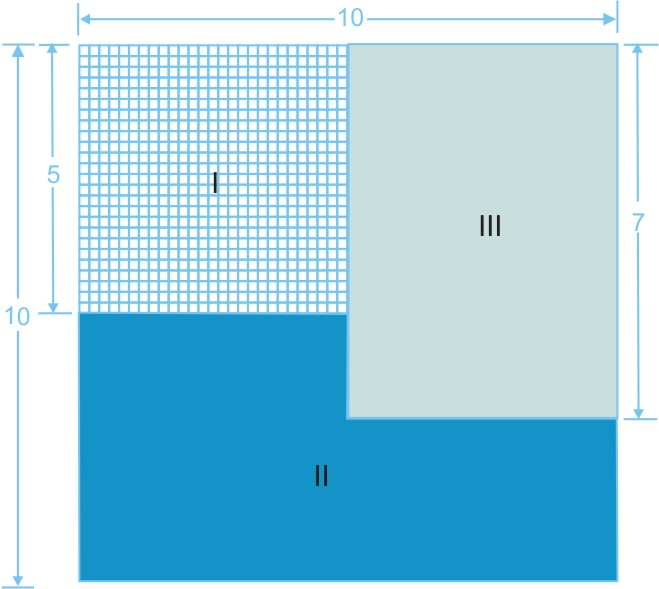#### In Fig. 8.8, what is the ratio of the areas of(a) shaded portion I to shaded portion II ?(b) shaded portion II to shaded portion III?(c) shaded portions I and II taken together and shaded portion III ?Area of shaded portion I = 5 x 5 =25 square units
Area of shaded portion III = 7 x 5 =35square units

Area of whole figure = 10 x 10 = 100 square units
Area of shaded portion II = Area of whole figure - Area of shaded portion I - Area of shaded portion III

= 100 - 25 - 35 = 40 square units

(a) Ratio of the areas of shaded portion I to shaded portion II

(b) Ratio of the areas of shaded portion II to shaded portion III

(c) Ratio of the areas of shaded portions I and II taken together and shaded portion III